# Critical Values: Find a Critical Value in Any Tail

Share on

Critical Values: Contents

## 1. What are Critical Values?

A critical value is a line on a graph that splits the graph into sections. One or two of the sections is the “rejection region“; if your test value falls into that region, then you reject the null hypothesis.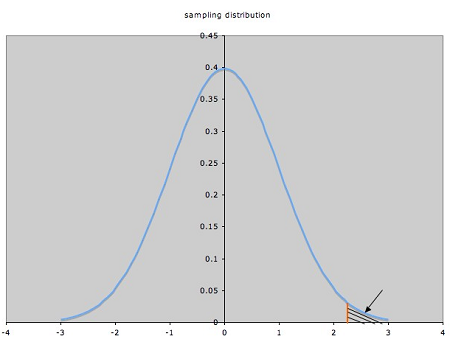A one tailed test with the rejection in one tail. The critical value is the red line to the left of that region.

Critical values come in all shapes and sizes, but the one you’ll come across first in statistics is the critical value of Z.

Watch the video for an overview of critical values:

Note: A critical number, used in calculus, is not the same thing as a critical value. Critical numbers are used in calculus to find points where a graph changes from increasing to decreasing, or vice-versa.

## 2.Critical Values of Z

The critical value of z is term linked to the area under the standard normal model. Critical values can tell you what probability any particular data point will have.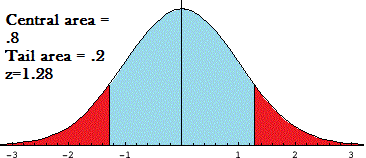The above graph of the normal distribution curve shows a critical value of 1.28. The graph has two parts:

A critical value of z is sometimes written as za, where the alpha level, a, is the area in the tail. For example, z.10 = 1.28.

## When are Critical values of z used?

A critical value of z (Z-score) is used when the sampling distribution is normal, or close to normal. Z-scores are used when the population standard deviation is known or when you have larger sample sizes. While the z-score can also be used to calculate probability for unknown standard deviations and small samples, in real life you’ll probabably use the t distribution to calculate these probabilities. That’s because you often don’t know the population variance (which is a requirement for using the z test).

## Other uses of z-scores

Every statistic has a probability, and every probability calculated for a sample has a margin of error. The critical value of z can also be used to calculate the margin of error.

## 3. Find Critical Values in Any Tail

Need help? Check out our tutoring page!

How you look up a critical value is very straightforward as long as you know if you have a left tailed test or right tailed test (or potentially, both).

## A. Find a critical value for a confidence level

Watch the video for an example:

Example question: Find a critical value for a 90% confidence level (Two-Tailed Test).

Step 1: Subtract the confidence level from 100% to find the α level: 100% – 90% = 10%.

Step 2: Convert Step 1 to a decimal: 10% = 0.10.

Step 3: Divide Step 2 by 2 (this is called “α/2”).
0.10 = 0.05. This is the area in each tail.

Step 4: Subtract Step 3 from 1 (because we want the area in the middle, not the area in the tail):
1 – 0.05 = .95.

Step 5: Look up the area from Step in the z-table. The area is at z=1.645. This is your critical value for a confidence level of 90%.

## B. Common confidence levels and their critical values

You don’t have to perform the above calculations every time. This list of z- critical values and their associated confidence levels were calculated using the above steps:

 Confidence Level Two Sided CV One Sided CV 90% 1.64 1.28 95% 1.96 1.65 99% 2.58 2.33

## C. Find Critical Values: Two-Tailed Test

Watch the video or read the steps below:

Example question: Find the critical value for alpha of .05.

Step 1: Subtract alpha from 1.

1 – .05 = .95

Step 2: Divide Step 1 by 2 (because we are looking for a two-tailed test).

.95 / 2 = .475

Step 3: Look at your z-table and locate the answer from Step 2 in the middle section of the z-table. The fastest way to do this is to use the find function of your browser (usually CTRL+F). In this example we’re going to look for .475, so go ahead and press CTRL+F, then type in .475.

Step 4: In this example, you should have found the number .4750. Look to the far left or the row, you’ll see the number 1.9 and look to the top of the column, you’ll see .06. Add them together to get 1.96. That’s the critical value!

Tip: The critical value appears twice in the z table because you’re looking for both a left hand and a right hand tail, so don’t forget to add the plus or minus sign! In our example you’d get ±1.96.

## D. Find a Critical Value: Right-Tailed Test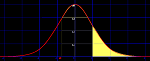Example question: Find a critical value in the z-table for an alpha level of 0.0079.

Step 1: Draw a diagram, like the one above. Shade in the area in the right tail.
This area represents alpha, α. A diagram helps you to visualize what area you are looking for (i.e. if you want an area to the right of the mean or the left of the mean).

Step 2: Subtract alpha (α) from 0.5.
0.5-0.0079 = 0.4921.

Step 3: Find the result from step 2 in the center part of the z-table.
The closest area to 0.4921 is 0.4922 at z = 2.42.

That’s it!

Like the explanation? Check out the Practically Cheating Statistics Handbook, which has hundreds more step-by-step solutions, just like this one!

## E. Find a Critical Value: Left-Tailed Test

Example question: find the critical value in the z-table for α = .012 (left-tailed test).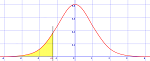Step 1: Draw a diagram, like the one above. Shade in the area in the left tail (because you’re looking for a critical value for a left-tailed test).
This area represents alpha, α .

Step 2: Subtract alpha (α) from 0.5.
0.5 – 0.012 = 0.488.

Step 3: Find the result from step 2 in the center part of the z-table.

The closest area to 0.488 is at z = 2.26. If you can’t find the exact area, just find the closest number and read the z value for that number.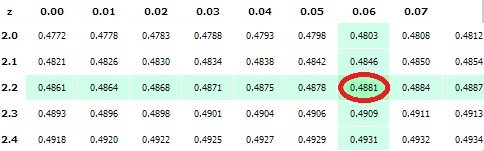Step 4: Add a negative sign to Step 3 (left-tail critical values are always negative).
-2.26.

That’s it!

Like the explanation? Check out our statistics how-to book, with a how-to for every elementary statistics problem type.

## 4. Critical Values and Working with Samples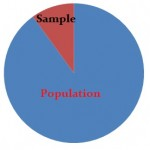Critical values are used in statistics for hypothesis testing. When you work with statistics, you’re working with a small percentage (a sample) of a population. For example, you might have statistics for voting habits from two percent of democratic voters, or five percent of students and their test results. Because you’re working with a fraction of the population and not the entire population, you can never be one hundred percent certain that your results reflect the actual population’s results. You might be 90 percent certain, or even 99 percent certain, but you can never be 100 percent certain. How accurate are your results? You can tell with hypothesis testing.

## 5. Types of Critical Values

Various types of critical values are used to calculate significance, including: t scores from student’s t-tests, chi-square, and z-tests. In each of these tests, you’ll have an area where you are able to reject the null hypothesis, and an area where you cannot. The line that separates these two regions is where your critical values are.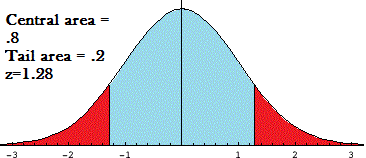In the above image, the critical values are at 1.28 or -1.28. The blue area is where you must accept the null hypothesis. The red areas are where you can reject the null hypothesis. How large these areas actually are (and what test you use) is dependent on many factors, including your chosen confidence level and your sample size.

## Significance Testing Example

Significance testing is used to figure out if your results differ from the null hypothesis. The null hypothesis is just an accepted fact about the population.

For example, your school may make a statistics course mandatory for nursing students because research has shown that patient outcomes improve when nurses have a statistics background. You might think that there’s no difference. Instead of trying to prove that there’s no difference, proper research techniques dictate that you’ll try to disprove the opposite — the null hypothesis, which in this case is that “patient outcomes improve when nurses have a statistics background.” In order to disprove, or reject, the null hypothesis, your research must pass a test of significance.

## 6. What does Significance Testing Tell Us?

Significance testing is used to calculate the probability that a relationship between two variables (like “taking a statistics class” and “improved patient outcomes”) is just due to chance. It helps to answer the question of whether you could duplicate your test results accurately in further research. By using probability and the normal curve, you can figure out what the chance is that your research is wrong.

Steps in Testing for Statistical Significance

1. State the Alternate Hypothesis.
2. State the Null Hypothesis.
3. Select a probability of error level (alpha level).
4. Select and compute the test for statistical significance (i.e. calculate a z-score.)
5. Interpret the results.

## References

Kenney, J. F. and Keeping, E. S. “Confidence Interval Charts.” §11.5 in Mathematics of Statistics, Pt. 1, 3rd ed. Princeton, NJ: Van Nostrand, pp. 167-169, 1962.

CITE THIS AS:
Stephanie Glen. "Critical Values: Find a Critical Value in Any Tail" From StatisticsHowTo.com: Elementary Statistics for the rest of us! https://www.statisticshowto.com/probability-and-statistics/find-critical-values/
---------------------------------------------------------------------------Need help with a homework or test question? With Chegg Study, you can get step-by-step solutions to your questions from an expert in the field. Your first 30 minutes with a Chegg tutor is free!